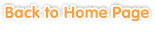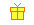Sample questions are shown below. Just click to start!• Chapter 1
Addition and Subtraction 3 and 4-Digit Numbers
•••
Subtraction with 3-Digit Numbers•• Chapter 2
Problem Solving: 3-4 Digit Addition and Subtraction• Chapter 3
Multiplying a Multiple-Digit Number with a 1-Digit Number
•
Mental Math•• Chapter 4
Dividing a Multiple-Digit Number with a 1-Digit Number
•
Division without Remainders•• Chapter 5
Multiplying a 2-Digit Number with a 2-Digit Number
•
Mental Math•• Chapter 6• Chapter 7
Fractions
••
Comparing Fractions•
Adding and Subtracting Fractions with Common Denominators• Chapter 8
Decimals
•
Basis of Decimals•
Comparing Decimals•• Chapter 9
Customary Measurement
•
Measuring Lengths in Inches•
Length Conversion (inches, feet, yards, and miles)•
Weight Conversion (ounces, pounds, and tons)• Chapter 10
Metric Measurement
•
Conversion (millimeters, centimeters, meters, and kilometers)•
Weight Conversion (Metric tons, grams, and kilograms)• Chapter 11
Time
•
Converting Time Units (hours, minutes, and seconds)•
24 Hour Clock••
Problem Solving: Time, Elapsed Time, and Calendar• Chapter 12
Geometry
•
Parallel and Perpendicular•